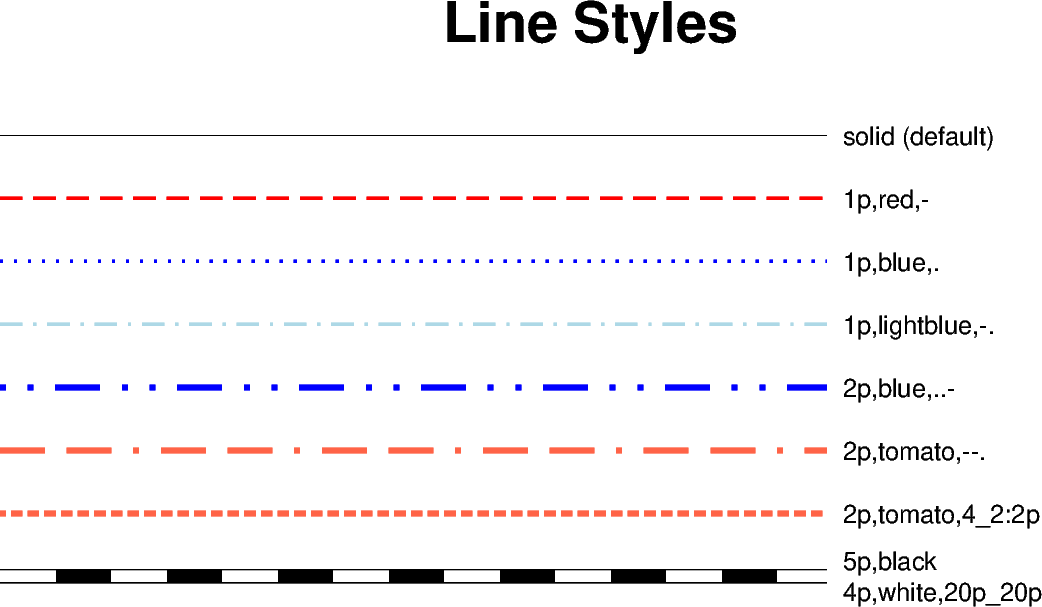# Line styles

The `pygmt.Figure.plot` method can plot lines in different styles. The default line style is a 0.25-point wide, black, solid line, and can be customized with the `pen` parameter.

A pen in GMT has three attributes: width, color, and style. The style attribute controls the appearance of the line. Giving `"dotted"` or `"."` yields a dotted line, whereas a dashed pen is requested with `"dashed"` or `"-"`. Also combinations of dots and dashes, like `".-"` for a dot-dashed line, are allowed.

For more advanced pen attributes, see the GMT cookbook https://docs.generic-mapping-tools.org/6.4/cookbook/features.html#wpen-attrib.```import numpy as np
import pygmt

# Generate a two-point line for plotting
x = np.array([0, 7])
y = np.array([9, 9])

fig = pygmt.Figure()
fig.basemap(region=[0, 10, 0, 10], projection="X15c/8c", frame="+tLine Styles")

# Plot the line using the default line style
fig.plot(x=x, y=y)
fig.text(x=x[-1], y=y[-1], text="solid (default)", justify="ML", offset="0.2c/0c")

# Plot the line using different line styles
for linestyle in [
"1p,red,-",  # dashed line
"1p,blue,.",  # dotted line
"1p,lightblue,-.",  # dash-dotted line
"2p,blue,..-",  # dot-dot-dashed line
"2p,tomato,--.",  # dash-dash-dotted line
# A pattern of 4-point-long line segments and 2-point-long gaps between
# segments, with pattern offset by 2 points from the origin
"2p,tomato,4_2:2p",
]:
y -= 1  # Move the current line down
fig.plot(x=x, y=y, pen=linestyle)
fig.text(x=x[-1], y=y[-1], text=linestyle, justify="ML", offset="0.2c/0c")

# Plot the line like a railway track (black/white).
# The trick here is plotting the same line twice but with different line styles
y -= 1  # move the current line down
fig.plot(x=x, y=y, pen="5p,black")
fig.plot(x=x, y=y, pen="4p,white,20p_20p")
fig.text(x=x[-1], y=y[-1], text="5p,black", justify="ML", offset="0.2c/0.2c")
fig.text(x=x[-1], y=y[-1], text="4p,white,20p_20p", justify="ML", offset="0.2c/-0.2c")

fig.show()
```

Total running time of the script: (0 minutes 3.189 seconds)

Gallery generated by Sphinx-Gallery